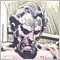Why calculations of period()_value are different? (over 30 minutes) What's Burg?19

//+------------------------------------------------------------------+
//|                                               check_Period().mq5 |
//|                      Copyright 2018, FIreFox,saboss,wmh,winstock |
//+------------------------------------------------------------------+
#property version   "1.00"
#property indicator_chart_window

ENUM_TIMEFRAMES TF={PERIOD_MN1,PERIOD_W1,PERIOD_D1,PERIOD_H4,PERIOD_H1,PERIOD_M30,PERIOD_M15,PERIOD_M5,PERIOD_M1};
//+------------------------------------------------------------------+
//| Custom indicator iteration function                              |
//+------------------------------------------------------------------+
int OnCalculate(const int rates_total,
const int prev_calculated,
const datetime &time[],
const double &open[],
const double &high[],
const double &low[],
const double &close[],
const long &tick_volume[],
const long &volume[],
{
//---
for(int i=0; i<9;i++) Alert(i,"   TF", string(i) ," =  ",TF[i]);
//--- return value of prev_calculated for next call
return(rates_total);
}
//+------------------------------------------------------------------+
//+------------------------------------------------------------------+
//| Custom indicator initialization function                         |
//+------------------------------------------------------------------+
int OnInit()
{
//--- indicator buffers mapping
//---
return(INIT_SUCCEEDED);
}193622

ssaboss:

Those numbers are correct. They do not represent the number of minutes within each period; rather, they are simply index numbers into the enum chosen by MetaQuotes.

Use EnumToString(TF[i]) to see the string representation of the time frame.

Use PeriodSeconds(TF[i]) to see the duration of the time frame.19

Dear Anthony Garot

Thanks to you for solving my problem.

The blessings of God

i changedd /w  PeriodSeconds(TF[i])  instead of TF[i]